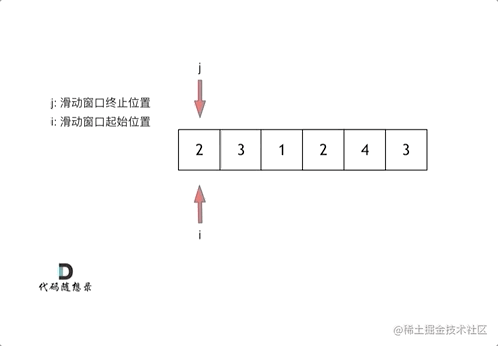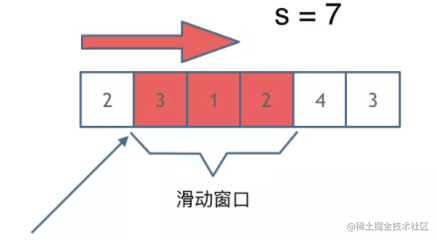数组常用算法之滑动窗口暴力解法

代码

class Solution {
public int minSubArrayLen(int target, int[] nums) {
int result = Integer.MAX_VALUE; // 最终的结果
int sum = 0; // 子序列的数值之和
int subLength = 0; // 子序列的长度
for (int i = 0; i < nums.length; i++) { // 设置子序列起点为i
sum = 0;
for (int j = i; j <  nums.length; j++) { // 设置子序列终止位置为j
sum += nums[j];
if (sum >= target) { // 一旦发现子序列和超过了s，更新result
subLength = j - i + 1; // 取子序列的长度
result = result < subLength ? result : subLength;
break; // 因为我们是找符合条件最短的子序列，所以一旦符合条件就break
}
}
}
// 如果result没有被赋值的话，就返回0，说明没有符合条件的子序列
return result == Integer.MAX_VALUE ? 0 : result;
}
}

• 时间复杂度：O(n^2)
• 空间复杂度：O(1)

滑动窗口算法• 窗口内是什么？
• 如何移动窗口的起始位置？
• 如何移动窗口的结束位置？滑动窗口的难点和精髓就在于在找到满足条件的窗口之后，前后两个指针怎么移动:

while (sum >= target) {
//计算满足条件的窗口长度
subLength = Math.min(subLength, right - left + 1);
//这里其实也可以分开写，sum先减去左边指针的值，然后左边指针往右移动
sum -= array[left++];
}
}

完整代码

class Solution {
public int minSubArrayLen(int target, int[] array) {
int length = array.length; int subLength = length + 1;
int sum = 0; int left = 0;
for (int right = 0; right < length; right++) {
sum += array[right];
while (sum >= target) {
subLength = Math.min(subLength, right - left + 1);
sum -= array[left++];
}
}
return subLength > length ? 0 : subLength;
}
}

复杂度

• 时间复杂度：O(n)
• 空间复杂度：O(1)

【参考】
【1】github.com/youngyangya…